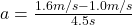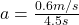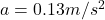## John runs 1.0 m/s at first, and then accelerates to 1.6 m/s during the course of 4.5 seconds. What is his average acceleration (in

Question

John runs 1.0 m/s at first, and then accelerates to 1.6 m/s during
the course of 4.5 seconds. What is his average acceleration (in
m/s2

in progress 0
5 months 2021-09-05T16:50:58+00:00 2 Answers 20 views 0

Explanation:

2. Answer: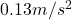Explanation: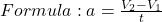Where;

a = acceleration

V2 = final velocity

V1 = initial velocity

t = time

If John runs 1.0 m/s first, we assume this is V1. He accelerates to 1.6 m/s; this is V2.